The recent releases of SAS 9.4 have featured major enhancements to the ODS statistical graphics procedures such as PROC SGPLOT. In fact, PROC SGPLOT (and the underlying Graph Template Language (GTL)) are so versatile and powerful that you might forget to consider whether you can create a graph automatically by using a SAS statistical procedure. For example, when you turn on ODS graphics (ODS GRAPHICS ON), SAS procedures create the following graphs automatically:

1. Many SAS regression procedures (and PROC PLM) create effect plots.
2. PROC SURVEYREG creates a hexagonal bin plot.
3. PROC REG creates heat maps when a scatter plot would suffer from overplotting.
4. PROC LOGISTIC creates odds-ratio plots.

### Let PROC FREQ visualize your two-way tables

Recently a SAS customer asked how to use PROC SGPLOT to produce a stacked bar chart. I showed him how to do it, but I also mentioned that the same graph could be produced with less effort by using PROC FREQ.

PROC FREQ is a workhorse procedure that can create dozens of graphs. For example, PROC FREQ can create a mosaic plot and a clustered bar chart to visualize frequencies and relative frequencies in a two-way table. Next time you use PROC FREQ, add the PLOTS=ALL option to the TABLES statement and see what you get!

One of my favorite plots for two-way categorical data is the stacked bar chart. As I told the SAS customer, it is simple to create a stacked bar chart in PROC FREQ. For example, the following statements create a horizontal bar chart that orders the categories by frequency:

```proc freq data=sashelp.Heart order=freq; tables weight_status*smoking_status / plots=freqplot(twoway=stacked orient=horizontal); run;```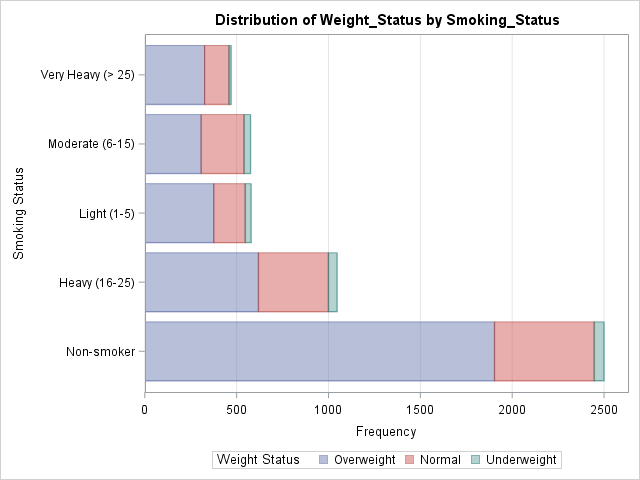See the documentation for the PLOTS= option in the TABLES statement for a description of all the plots that PROC FREQ can create. PROC FREQ creates many plots that are associated with a particular analysis, such as the "deviation plot," which shows the relative deviations between the observed and expected counts when you request a chi-square analysis of a one-way table.

### Do you need a highly customized graph?

The ODS graphics in SAS are designed to create many—but not all—of the visualizations that are relevant to an analysis. Some attributes of a graph (for example, the title and the legend placement) are determined by a stored template and can't be modified by using the procedure syntax. Advanced GTL gurus might want to learn how to edit the ODS templates. Less ambitious users might choose to use a statistical procedure to automatically create graphs during data exploration and modeling, but then use PROC SGPLOT to create the final graph for a report.

For example, the following call to PROC FREQ writes the two-way frequency counts to a SAS data set. From the data you can create graphs that are similar to the one that PROC FREQ creates, but you can change the order and colors of the bars, alter the placement of the legend, add text, and more. The following call to PROC SGPLOT shows one possibility. Click on the graph to see the full-size version.

```proc freq data=sashelp.heart order=freq noprint; tables smoking_status*weight_status / out=FreqOut(where=(percent^=.)); run;   ods graphics /height=500px width=800px; title "Counts of Weight Categories by Smoking Status"; proc sgplot data=FreqOut; hbarparm category=smoking_status response=count / group=weight_status seglabel seglabelfitpolicy=none seglabelattrs=(weight=bold); keylegend / opaque across=1 position=bottomright location=inside; xaxis grid; yaxis labelpos=top; run;```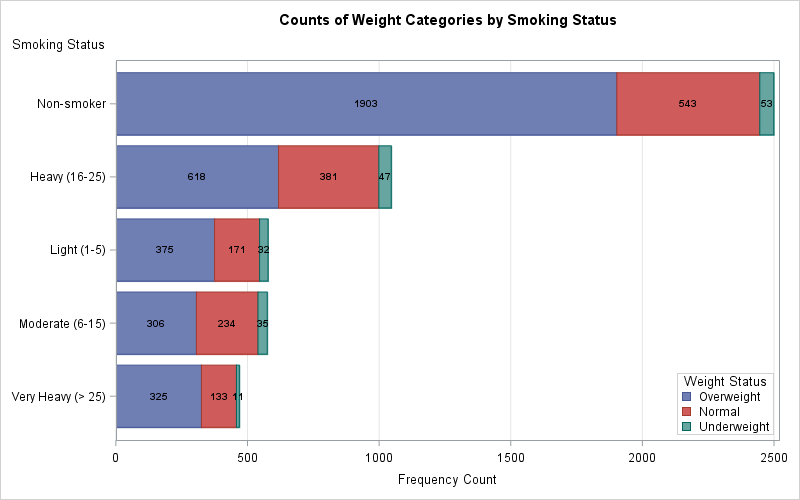### Conclusions

If you want to create a stacked bar chart or some other visualization of a two-way table, you might be tempted to immediately start using PROC SGPLOT. The purpose of this article is to remind you that SAS statistical procedures, including PROC FREQ, often create graphs as part of their output. Even if a statistical procedure cannot provide all the bells and whistles of PROC SGPLOT, it often is a convenient way to visualize your data during the preliminary stages of an analysis. If you need a highly customized graph for a final report, the SAS procedure can output the data for the graph. You can then use PROC SGPLOT or the GTL to create a customized graph.

The post Let PROC FREQ create graphs of your two-way tables appeared first on The DO Loop.Finding nearest neighbors is an important step in many statistical computations such as local regression, clustering, and the analysis of spatial point patterns. Several SAS procedures find nearest neighbors as part of an analysis, including as PROC LOESS, PROC CLUSTER, PROC MODECLUS, and PROC SPP. This article shows how to find nearest neighbors for every observation directly in SAS/IML, which is useful if you are implementing certain algorithms in that language.

### Compute the distance between observations

Let's create a sample data set. The following DATA step simulates 100 observations that are uniformly distributed in the unit square [0,1] x [0,1]:

```data Unif; call streaminit(12345); do i = 1 to 100; x = rand("Uniform"); y = rand("Uniform"); output; end; run;```

I have previously shown how to compute the distance between observations in SAS by using PROC DISTANCE or the DISTANCE function in SAS/IML. The following statements read the data into a SAS/IML matrix and computes the pairwise distances between all observations:

```proc iml; use Unif; read all var {x, y} into X; close; D = distance(X); /* N x N distance matrix */```

The D matrix is a symmetric 100 x 100 matrix. The value D[i,j] is the Euclidean distance between the ith and jth rows of X. An easy way to look for the nearest neighbor of observation i is to search the ith row for the column that contains smallest distance. Because the diagonal elements of D are all zero, a useful trick is to change the diagonal elements to be missing values. Then the smallest value in each row of D corresponds to the nearest neighbor.

You can use the following statements assigns missing values to the diagonal elements of the D matrix. You can then use the SAS/IML subscript reduction operators to find the minimum distance in each row:

```diagIdx = do(1, nrow(D)*ncol(D), ncol(D)+1); /* index diagonal elements */ D[diagIdx] = .; /* set diagonal elements */   dist = D[ ,><]; /* smallest distance in each row */ nbrIdx = D[ ,>:<]; /* column of smallest distance in each row */ Neighbor = X[nbrIdx, ]; /* coords of closest neighbor */```

### Visualizing nearest neighbors

If you write the nearest neighbors and distances to a SAS data set, you can use the VECTOR statement [LINK] in PROC SGPLOT to draw a vector that connects each observation to its nearest neighbor. The graph indicates the nearest neighbor for each observation.

```Z = X || Neighbor || dist; create NN_Unif from Z[c={"x" "y" "xc" "yc" "dist"}]; append from Z; close; QUIT;   title "Nearest Neighbors for 100 Uniformly Distributed Points"; proc sgplot data=NN_Unif aspect=1; label dist = "Distance to Nearest Neighbor"; scatter x=x y=y / colorresponse=dist markerattrs=(symbol=CircleFilled); vector x=xc y=yc / xorigin=x yorigin=y transparency=0.5 colorresponse=dist; /* COLORRESPONSE= requires 9.4m3 for VECTOR */ xaxis display=(nolabel); yaxis display=(nolabel); run;```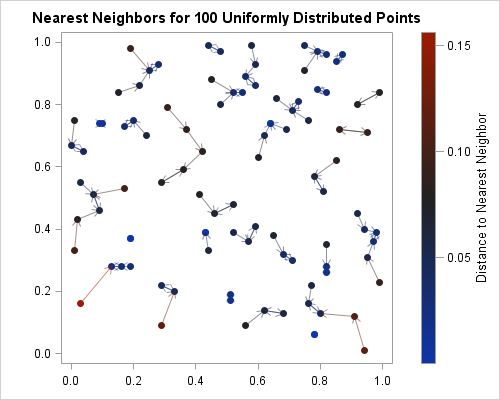### Alternative ways to compute nearest neighbors

If you don't have SAS/IML but still want to compute nearest neighbors, you can use PROC MODECLUS. The NEIGHBOR option on the PROC MODECLUS statement produces a table that gives the observation number (or ID value) of nearest neighbors. For example, the following statements produce the observation numbers for the nearest neighbors:

```ods select neighbor; /* Use K=p to find nearest p-1 neighbors */ proc modeclus data=Unif method=1 k=2 Neighbor; /* k=2 for nearest nbrs */ var x y; run;```

To get the coordinates of the nearest neighbor, you can create a variable that contains the observation numbers and then use an ID statement to include that ID variable in the PROC MODECLUS output. You can then look up the coordinates. I omit the details.

### Why stop at one? A SAS/IML module for k nearest neighbors

You can use the ideas in the earlier SAS/IML program to write a program that returns the indices (observation numbers) of the k closest neighbors for k ≥ 1. The trick is to replace the smallest distance in each row with a missing value and then repeat the process of finding the smallest value (and column) in each row. The following SAS/IML module implements this computation:

```proc iml; /* Compute indices (row numbers) of k nearest neighbors. INPUT: X an (N x p) data matrix k specifies the number of nearest neighbors (k>=1) OUTPUT: idx an (N x k) matrix of row numbers. idx[,j] contains the row numbers (in X) of the j_th closest neighbors dist an (N x k) matrix. dist[,j] contains the distances between X and the j_th closest neighbors */ start NearestNbr(idx, dist, X, k=1); N = nrow(X); p = ncol(X); idx = j(N, k, .); /* j_th col contains obs numbers for j_th closest obs */ dist = j(N, k, .); /* j_th col contains distance for j_th closest obs */ D = distance(X); D[do(1, N*N, N+1)] = .; /* set diagonal elements to missing */ do j = 1 to k; dist[,j] = D[ ,><]; /* smallest distance in each row */ idx[,j] = D[ ,>:<]; /* column of smallest distance in each row */ if j < k then do; /* prepare for next closest neighbors */ ndx = sub2ndx(dimension(D), T(1:N)||idx[,j]); D[ndx] = .; /* set elements to missing */ end; end; finish;```

You can use this module to compute the k closest neighbors for each observation (row) in a data matrix. For example, the following statements compute the two closest neighbors for each observation. The output shows a few rows of the original data and the coordinates of the closest and next closest observations:

```use Unif; read all var {x, y} into X; close;   k=2; run NearestNbr(nbrIdx, dist, X, k); Near1 = X[nbrIdx[,1], ]; /* 1st nearest neighbors */ Near2 = X[nbrIdx[,2], ]; /* 2nd nearest neighbors */```In summary, this article defines a short module in the SAS/IML language that you can use to compute the k nearest neighbors for a set of N numerical observations. Notice that the computation builds an N x N distance matrix in RAM, so this matrix might consume a lot of memory for large data sets. For example, the distance matrix for a data set with 16,000 observations requires about 1.91 GB of memory. For larger data sets, you might prefer to use PROC DISTANCE or PROC MODECLUS.

The post Compute nearest neighbors in SAS appeared first on The DO Loop.One of the strengths of the SGPLOT procedure in SAS is the ease with which you can overlay multiple plots on the same graph. For example, you can easily combine the SCATTER and SERIES statements to add a curve to a scatter plot.

However, if you try to overlay incompatible plot types, you will get an error message that says
`ERROR: Attempting to overlay incompatible plot or chart types.`
For example, a histogram and a series plots are not compatible in PROC SGPLOT, so you need to use the Graphics Template Language (GTL) to overlay a custom density estimate on a histogram.

A similar limitation exists for bar charts in PROC SGPLOT: you cannot specify the VBAR and SERIES statements in a single call. However, in SAS 9.4m3 you can overlay a curve and a bar chart by using the new the VBARBASIC and the HBARBASIC statements. These statements create a bar chart that is compatible with basic plots such as scatter plots, series plots, and box plots.

### Overlay a curve on a bar chart in SAS

In most situations it doesn't make sense to overlay a continuous curve on a discrete bar chart, which is why the SG routines have the concept of compatible plot types. However, there is a canonical example in elementary statistics that combines continuous and discrete data: the normal approximation to the binomial distribution.Recall that if X is the number of successes in n independent trials for which the probability of success is p, then X is binomially distributed: X ~ Binom(n, p). A well-known rule says that if np > 5 and n(1-p) > 5, then the binomial distribution is approximated by a normal distribution with mean np and standard deviation sqrt(np(1-p)).

This rule is often illustrated by overlaying the continuous normal PDF on a bar chart that shows the binomial distribution, as shown to the left. To create this plot, I used the VBARBASIC statement to create the bar chart. Because the VBARBASIC statement creates a "basic plot," you can combine it with another basic plot, such as the line plot created by using a SERIES statement. For fun, I used an INSET statement to overlay a box of parameter values for the graph. The graph shows that the binomial probability at j is approximated by the area under the normal density curve on the interval [j-0.5, j+0.5].

The following SAS statements use the PDF function to evaluate the binomial probabilities and the normal density for the graph. The values for μ and σ are stored in macro variables for later use.

```%let p = 0.25; /* probability of success */ %let n = 25; /* number of trials */ data Binom; n = &n; p = &p; q = 1 - p; mu = n*p; sigma = sqrt(n*p*q); /* parameters for the normal approximation */ Lower = mu-3.5*sigma; /* evaluate normal density on [Lower, Upper] */ Upper = mu+3.5*sigma;   /* PDF of normal distribution */ do t = Lower to Upper by sigma/20; Normal = pdf("normal", t, mu, sigma); output; end;   /* PMF of binomial distribution */ t = .; Normal = .; /* these variables are not used for the bar chart */ do j = max(0, floor(Lower)) to ceil(Upper); Binomial = pdf("Binomial", j, &p, &n); output; end; call symput("mu", strip(mu)); /* store mu and sigma in macro variables */ call symput("sigma", strip(round(sigma,0.01))); label Binomial="Binomial Probability" Normal="Normal Density"; keep t Normal j Binomial; run;```

The preceding DATA step evaluates the Binom(15, 0.25) probability for the integers j=0, 1, ..., 14. It evaluates the N(6.25, 2.17) PDF on the interval [-1.3, 13.8]. The following call to PROC SGPLOT uses the VBARBASIC statement to overlay the bar chart and the density curve:

```title "Binomial Probability and Normal Approximation"; proc sgplot data=Binom; vbarbasic j / response=Binomial barwidth=1; /* requires SAS 9.4M3 */ series x=t y=Normal / lineattrs=GraphData2(thickness=2); inset "n = &n" "p = &p" "q = %sysevalf(1-&p)" "(*ESC*){unicode mu} = np = &mu" /* use Greek letters */ "(*ESC*){unicode sigma} = sqrt(npq) = &sigma" / position=topright border; yaxis label="Probability"; xaxis label="x" integer type=linear; /* force TYPE=LINEAR */ run;```

The TYPE=LINEAR option on the XAXIS statement tells the horizontal axis to use interval tick marks. The BARWIDTH=1 option on the VBARBASIC statement makes the bar chart look more like a histogram by eliminating the gaps between bars. The graph is shown at the top of this section.

### Alternative visualization: The needle plot

If you are content to show only the height of the binomial probability mass function (PMF), you can use an alternative visualization. The following graph shows a needle plot (the binomial PMF) overlaid with a normal PDF. This visualization does not require 9.4M3. The SGPLOT statements are the same as before, except the binomial probabilities are represented by using the NEEDLE statement: needle x=j y=Binomial / markers;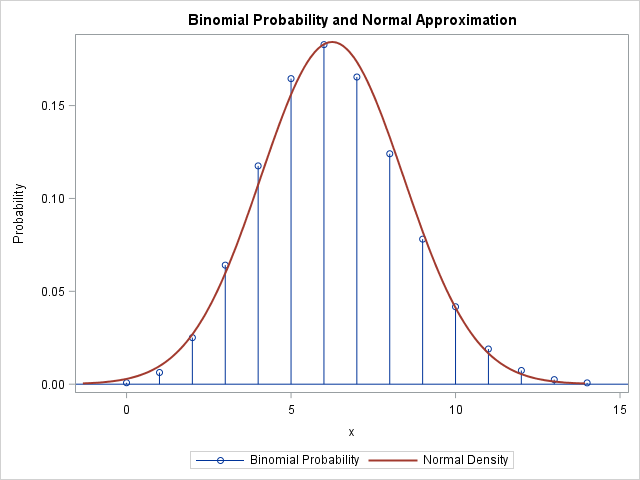tags: 14.1, 9.4, Statistical Graphics

The post Overlay a curve on a bar chart in SAS appeared first on The DO Loop.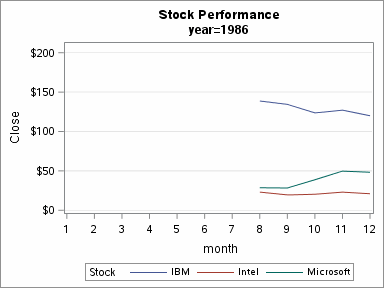It is easy to use PROC SGPLOT and BY-group processing to create an animated graph in SAS 9.4. Sanjay Matange previously discussed how to create an animated plot in SAS 9.4, but he used a macro loop to call PROC SGPLOT many times.

It is often easier to use the BY statement in SAS procedures to create many graphs. Someone recently asked me how I created an animation that shows level sets of a contour plot. This article explains how to create an animation by using the BY statement in PROC SGPLOT.

An animation requires that you create a sequence of images. In SAS 9.4, you can create an animated GIF by using the ODS PRINTER destination. ODS does not care how the images are generated. They can be created by a macro loop. Or, as shown below, they can be generated by using the BY statement in PROC SGPLOT, SGRENDER, or any other procedure in SAS.

As an example, I will create the graph at the top of this article, which shows the annual time series for the stock price of three US companies for 20 consecutive years. The data are contained in the Sashelp.Stocks data set. The following DATA step adds two new variables: Year and Month. The data are then sorted according to Date, which also sorts the data by Year.

```data stocks; set sashelp.stocks; Month = month(date); /* 1, 2, 3, ..., 12 */ Year = year(date); /* 1986, 1987, ..., 2005 */ run;   proc sort data=stocks; by date; run;```

I will create an animation that contains 20 frames. Each frame will be a graph that shows the stock performance for the three companies in a particular year. You can use PROC MEANS to discover that the stock prices are within the range [10, 210], so that range is used for the vertical axis:

```ods graphics / imagefmt=GIF width=4in height=3in; /* each image is 4in x 3in GIF */ options papersize=('4 in', '3 in') /* set size for images */ nodate nonumber /* do not show date, time, or frame number */ animduration=0.5 animloop=yes noanimoverlay /* animation details */ printerpath=gif animation=start; /* start recording images to GIF */ ods printer file='C:AnimGifByGroupAnim.gif'; /* images saved into animated GIF */   ods html select none; /* suppress screen output */ proc sgplot data=stocks; title "Stock Performance"; by year; /* create 20 images, one for each year */ series x=month y=close / group=stock; /* each image is a time series */ xaxis integer values=(1 to 12); yaxis min=10 max=210 grid; /* set common vertical scale for all graphs */ run; ods html select all; /* restore screen output */   options printerpath=gif animation=stop; /* stop recording images */ ods printer close; /* close the animated GIF file */```

The BY statement writes a series of images. They are placed into the animated GIF file that you specify on the FILE= option in the ODS PRINTER statement.

A few tricks are worth mentioning:
• Use the ODS GRAPHICS statement to specify the size of the image in some physical measurement (inches or centimeters). On the OPTIONS statement, specify those same measurements.
• You can control the animation by using SAS system options. To create an animated GIF, use OPTIONS PRINTERPATH=GIF ANIMATION=START before you generate the image files. Use OPTIONS PRINTERPATH=GIF ANIMATION=STOP after you generate the image files.
• Use the ANIMDURATION= option to specify the time interval (in seconds) that each frame appears. Typical values are 0.1 to 0.5.
• Use the ANIMLOOP= option to specify whether the animation should repeat after reaching the end
• Use ODS HTML SELECT NONE to prevent the animation frames from appearing in your HTML output. (If you use the LISTING output, replace "HTML" with "LISTING.")
• By default, the levels of the BY group are automatically displayed in the TITLE2 field of the graph. You can turn off this behavior by specifying the NOBYLINE option. You can use the expression #BYVAL(year) in a TITLE or FOOTNOTE statement to incorporate the BY-group level into a title or footnote.

You can use a browser to view the image. As I did in this blog post, you can embed the image in a web page.

tags: 9.4, Statistical Graphics

The post Create an animation with the BY statement in PROC SGPLOT appeared first on The DO Loop.You will never find me walking under a ladder, spilling salt without then throwing a pinch over my shoulder, or letting a penny remain on the sidewalk; I'm incredibly superstitious. In general I consider the number 13 to be unlucky. I'm not quite in triskadekaphobia territory, but I'm not too […]Compared with SAS 9.3, the latest SAS 9.4 introduced a few new procedures for the BASE and STAT components: 7 new procedures for BASE 9.4 and 4 for STAT 12.3. 6 high-performance procedures (thanks to Dr. Wicklin's correction).
New in BASE 9.4New in STAT 12.1New in STAT 12.3
DS2QUANTLIFEHPLOGISTIC
JSONQUANTSELECTHPLMIXED
PRESENVSTDRATEHPNLMOD
STREAMHPREG
FEDSQLHPSPLIT
AUTHLIB
DS2 is a new SAS proprietary programming language that is appropriate for advanced data manipulation.It is exciting to see the emergence of DS2 and FEDSQL. According to SAS 9.4 DS2 Language Reference,
DS2 is a SAS programming language that is appropriate for advanced data manipulation
Contrary to the thought I had last year, DS2 or `PROC DS2` is not a complied language. It seems more like a wrapper of PROC FEDSQL, which combines the capacity of SQL and the original DATA Step together. Therefore, DS2 includes many SQL's features such as subquery.
``data class;   set sashelp.class;run;proc datasets nolist;   delete _:;quit;proc ds2 stimer;   data _test1;      dcl varchar(6) gender;      method run();         set {select name, sex from class where age > 12};         if sex = 'M' then gender = 'Male';         else gender = 'Female';      end;   enddata;   run;quit;``
The functionality is equivalent to the SQL syntax in SAS below.
``proc sql stimer;   create table _test2 as   select *, case when sex = 'M' then 'Male'         else 'Female'      end as gender   from (select name, sex from class where age > 12);quit;``
Additionally, DS2 supports the concept of transaction in SQL. The `run` statement in DS2 is equal to the `COMMIT` statement in SQL, while `run cancel` statement is comparable to SQL's`ROLLBACK` statement.
In conclusion, with DS2, SAS is leaning toward RDBMS in how to understand and deal with data.